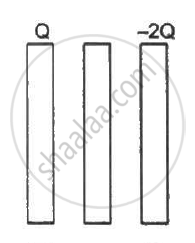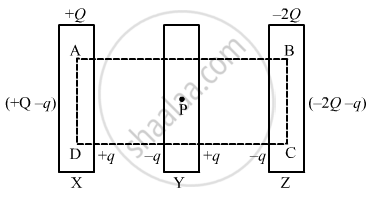Department of Pre-University Education, Karnataka course PUC Karnataka Science Class 12
Share

# Three identical metal plates with large surface areas are kept parallel to each other as shown in the following figure - Physics

ConceptElectric Field Introduction of Electric Field

#### Question

Three identical metal plates with large surface areas are kept parallel to each other as shown in the following figure. The leftmost plate is given a charge Q, the rightmost a charge −2Q and the middle one is kept neutral. Find the charge appearing on the outer surface of the rightmost plate.#### SolutionConsider the Gaussian surface as shown in the figure.

Let the charge on the outer surface of the left-most plate be q. Thus, the charges on the plates are distributed as shown in the following diagram.

The net field at point P due to all the induced charges must be zero, as it is lying inside the metal surface.

Let the surface area of the plates be A.

Electric field at point P due to the charges on plate X:-

Due to charge (+ Q -q) is ("Q" -"q")/(2"A"∈_0)  in the right direction

Due to charge (+q) is "q"/(2"A"∈_0) in the right direction

Electric field at point P due to charges on plate Y:-

Due to charge (-q) is "q"/(2"A"∈_0) in the right direction

Due to charge (+q) is "q"/(2"A"∈_0) in the left direction.

Electric field at point P due to charges on plate Z:-

Due to charge (-q) is "q"/(2"A"∈_0) in the right direction.

Due to charge (-2Q+q) is (2"Q"-"q")/(2"A"∈_0) in the right direction

The net electric field at point P:-

("Q"-"q")/(2"A"∈_0)+"q"/(2"A"∈_0) -"q"/(2"A"∈_0) - "q"/(2"A"∈_0) + "q"/(2"A"∈_0) + (2"Q"-"q")/(2"A"∈_0) = 0

("Q"-"q")/(2"A"∈_0) + (2"Q" -"q")/(2"A"∈_0) = 0

Q - q + 2Q - q = 0

3Q - q = 0

"q" =(3"Q")/2

Thus, the charge on the outer plate of the right-most plate = -2"Q" +"q" = -2"Q"+ (3"Q")/2 = -"Q"/2

Is there an error in this question or solution?

#### APPEARS IN

Solution Three identical metal plates with large surface areas are kept parallel to each other as shown in the following figure Concept: Electric Field - Introduction of Electric Field.
S